Computes the value of a pixel according to its spatial position.

## Syntax and Parameters

mt_lutspa (clip, string "mode", bool "relative", bool "biased", string "expr", string "yExpr", string "uExpr", string "vExpr", int "Y", int "U", int"V", string "chroma", int "offX", int "offY", int "w" int "h")

clip   =
The clip is used as a template (for the frame count, the width, the height and the colorspace).

string  mode = "relative"
mode controls how the pixels coordinates are transformed into a value. It overrides the legacy parameters, relative and biased.
Possible values for mode are :
• "absolute" : x and y go from 0 to width - 1 and height - 1. Equivalent to relative = false.
• "relative", or "relative opened", or "relative exclusive" : x and y go from 0 to 1 (excluded). Equivalent to relative = true, biased = true.
• "relative closed", or "relative inclusive" : x and y go from 0 to 1 (included). Equivalent to relative = true, biased = false.

bool  relative = true
relative, defaulted to true, indicates whether x & y should be absolute (x ranging from 0 to the clip's width, y from 0 to the height), or relative (x & y ranging from 0 to 1).

bool  biased = true
biased is used only when relative is true, and indicates whether x and y range from 0 to 1 included (biased = false) or excluded (biased = true).

string  expr = "x"
string  yExpr = "x"
string  uExpr = "x"
string  vExpr = "x"
It computes the value of each pixels according to its coordinates and the given lut. Variable x and y in the lut corresponds respectively to absciss and ordinate of the pixel.

int  Y = 3
int  U = 1
int  V = 1
These three values describe the actual processing mode that is to be used on each plane / channel. Here is how the modes are coded :
• x = -255...0 : all the pixels of the plane will be set to -x.
• x = 1 : the plane will not be processed. That means the content of the plane after the filter is pure garbage.
• x = 2 : the plane of the first input clip will be copied.
• x = 3 : the plane will be processed with the processing the filter is designed to do.

string  chroma = ""
When defined, the value contained in this string will overwrite the U and V processing modes.
This is a nice addition proposed by mg262 that makes the filter more user friendly. Allowed values for chroma are:
• "process" : set u = v = 3.
• "copy" or "copy first" : set u = v = 2.
• "xxx", where xxx is a number : set u = v = -xxx.

int  offX = 0
int  offY = 0
offX and offY are the top left coordinates of the box where the actual processing shall occur. Everything outside that box will be garbage.

int  w = -1
int  h = -1
w and h are the width and height of the processed box. -1 means that the box extends to the lower right corner of the video. That also means that default settings are meant to process the whole picture.

## Examples

For the following examples lets assume our input clip is: `BlankClip(width=128, height=128, pixel_type="Y8")`

• `mt_lutspa(mode="absolute", expr="x 32 % 16 < 0 255 ?")`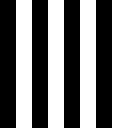• `mt_lutspa(expr="x 2 ^ y 2 ^ + 2 / 0.5 ^ 256 *")`
• `mt_lutspa(mode="relative", expr="y 32 480 / - 82 480 / / pi * sin 128 * 128 +")`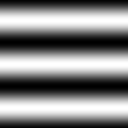• `mt_lutspa(mode="relative", expr="x y + 256 * 2 /")`
• `mt_lutspa(relative=false, expr="y 5 % 0.01 < 55 y 5 % 1.1 < 200 y 5 % 3.9 > 200 255 ? ? ?")`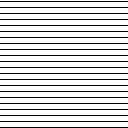• `mt_lutspa("absolute", expr="y 800 >= y 1000 <= & 255 y 800 y 1050 < & y 1050 – abs 5.1 * 0 ? ? ?")`
• `mt_lutspa(relative=false, expr="y 6 % 0.01 < 0 255 ?")`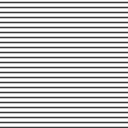• `mt_lutspa(mode="relative", expr="x 255 *")`
• `mt_lutspa(mode="absolute", expr="x 32 % 16 < 0 1 ? y 32 % 16 < 0 1 ? + 1 == 0 255 ?")`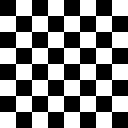• `mt_lutspa(mode="relative", expr="x 1 2 / - abs y 1 2 / - abs + 256 *")`
• `mt_lutspa(relative=false, expr="x 16 % 0 == y 16 % 0 == | 255 0 ?")`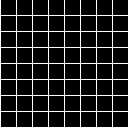• `mt_lutspa(mode="relative", expr="x y 1 x - 1 y - * * * 4096 *")`